## Stochastic Matrix

A Stochastic matrix is the transition matrix for a finite Markov Chain, also called a Markov Matrix. Elements of the matrix must be Real Numbers in the Closed Interval [0, 1].

A completely independent type of stochastic matrix is defined as a Square Matrix with entries in a Field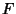such that the sum of elements in each column equals 1. There are two nonsingular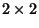Stochastic Matrices over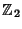(i.e., the integers mod 2),There are six nonsingular stochastic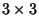Matrices over,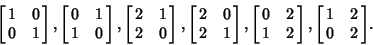In fact, the set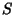of all nonsingular stochastic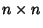matrices over a Fieldforms a Group under Matrix Multiplication. This Group is called the Stochastic Group.

Poole, D. G. The Stochastic Group.'' Amer. Math. Monthly 102, 798-801, 1995.